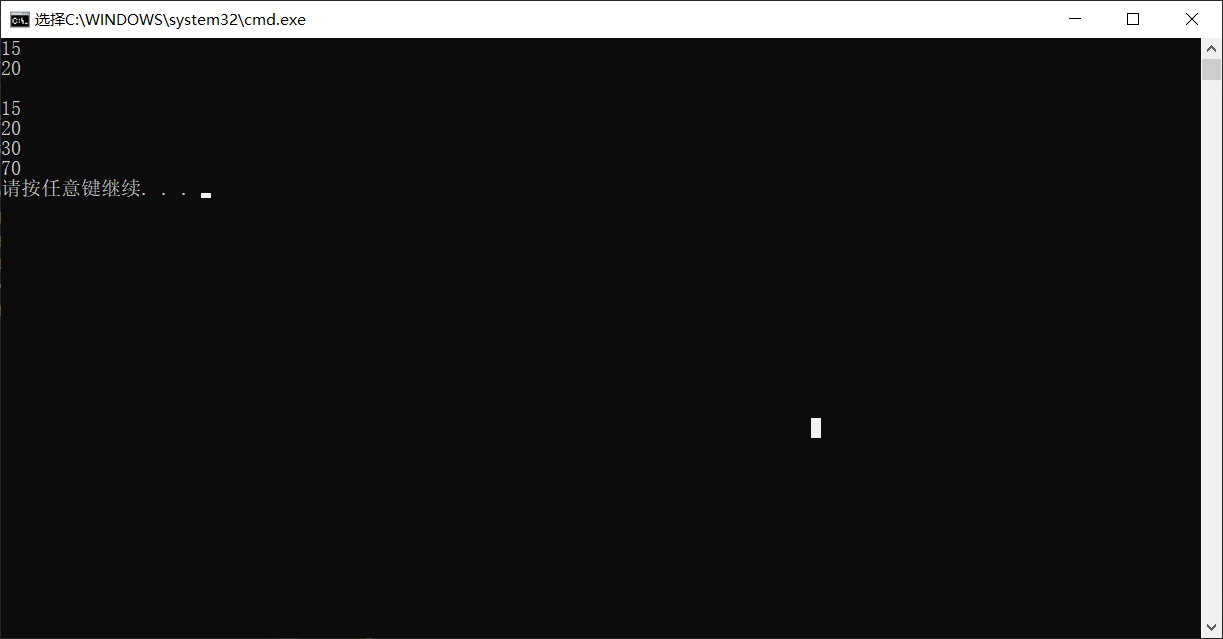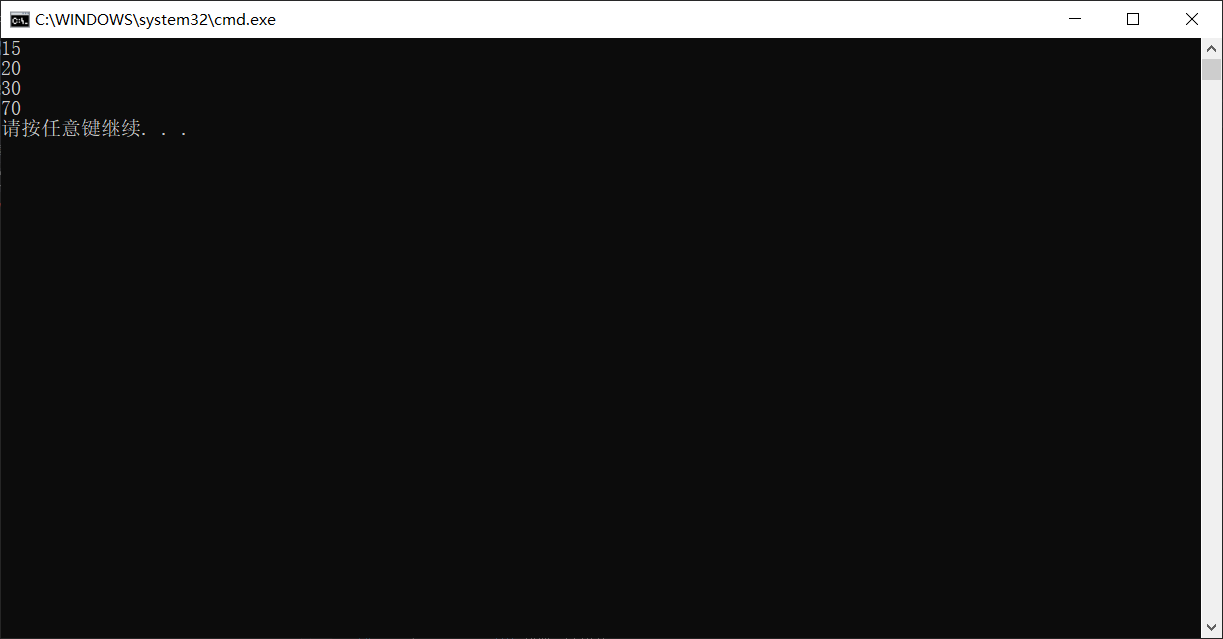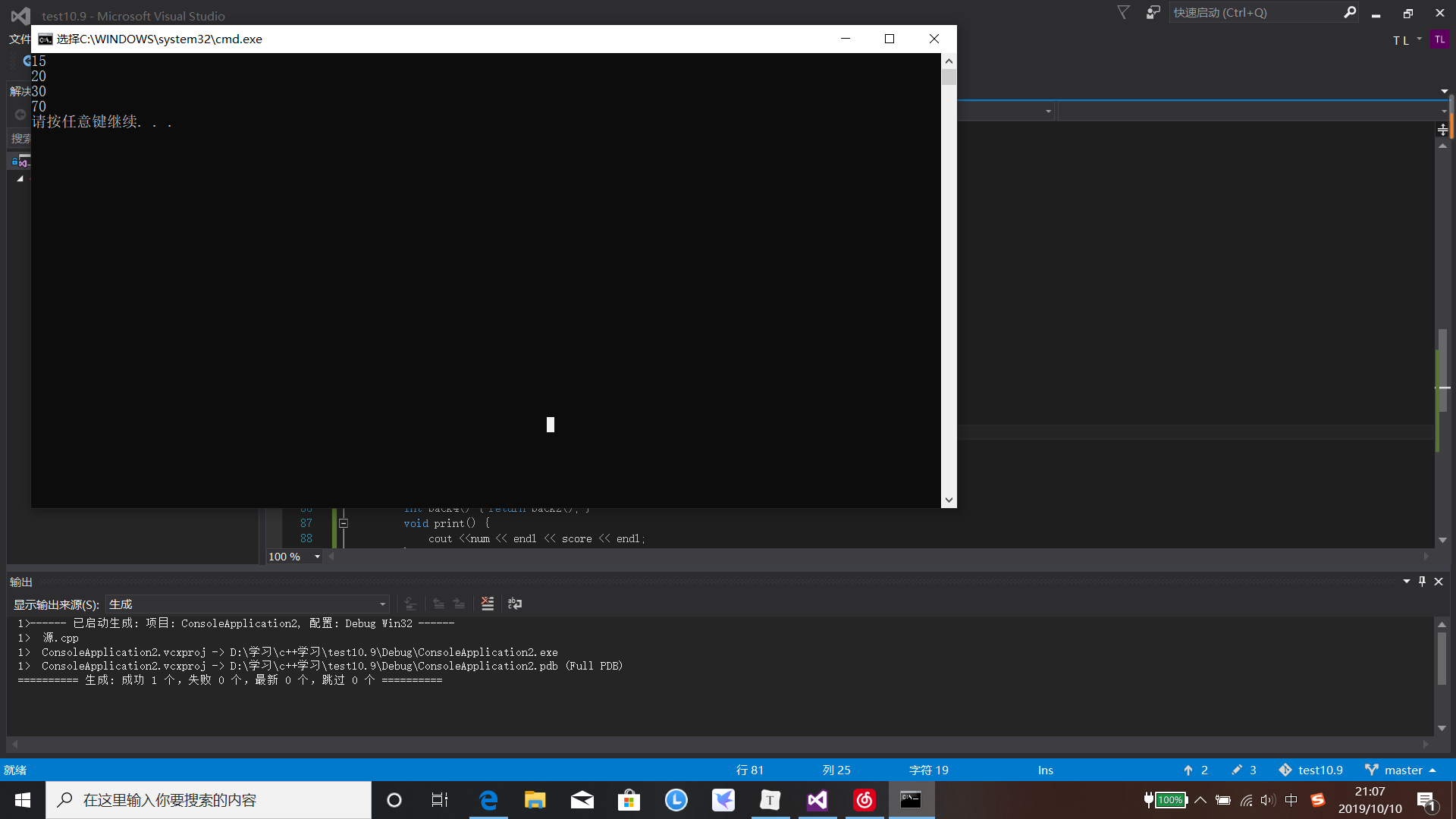# C++第四次作业：继承

#include<iostream>
using namespace std;
class person {
private: int id, age;
public:
person() { id = 15; age = 20; }
void show() {
cout << id << endl << age << endl<<endl;
}
};
class student {

private: int id, age;
int num, score;
public:  student() { id = 15; age = 20; num = 30; score = 70; }
void print() {
cout << id << endl << age << endl << num << endl << score << endl;
}

};
int main()
{
person a;
student b;
a.show();
b.print();
}## public继承

#include<iostream>
using namespace std;
class person {
private: int id, age;
public:
void set(int a, int b) { this->id = a; this->age = b; }
void show() {
cout << id << endl << age << endl;
}
};
class student:public person {
private:
int num, score;
public:  student() {  num = 30; score = 70; }
void print() {
cout << num << endl << score << endl;
}

};
int main()
{

student a;
a.set(15, 20);
a.show();
a.print();
}## protected继承

#include<iostream>
using namespace std;
class person {
private: int id, age;
public:
person() { id=10; age=20; }
void show() {
cout << id << endl << age << endl;
}
int back() {   return id; }
int back2() {  return age; }

};
class student:protected person {
private:
int num, score;
public:  student() {  num = 30; score = 70; }

int back3() { return back(); }
int back4() { return back2(); }
void print() {
cout <<num << endl << score << endl;
}

};
int main()
{
int c,d;

student a;
c = a.back3();
d = a.back4();
cout << d<<endl<<c << endl;
a.print();
}## private继承

private继承后基类中公有成员与保护成员都以私有成员的身份出现在派生类中，而基类的私有成员也是不可以被派生类所访问的，所以私有继承后，派生类的成员可以直接访问保护与公有成员，但是无论通过派生类的对象还是成员都无法直接访问基类私有成员

#include<iostream>
using namespace std;
class person {
private: int id, age;
public:
person() { id=10; age=20; }
void show() {
cout << id << endl << age << endl;
}
int back() {   return id; }
int back2() {  return age; }

};
class student:private person {
private:
int num, score;
public:  student() {  num = 30; score = 70; }

int back3() { return back(); }
int back4() { return back2(); }
void print() {
cout <<num << endl << score << endl;
}

};
int main()
{
int c,d;

student a;
c = a.back3();
d = a.back4();
cout << d<<endl<<c << endl;
a.print();
}



## 作业总结

1：对于公有继承来说派生类可以访问基类成员中的公有成员以及保护成员，并且基类成员对派生类对象的可见性对派生类对象来说，基类的公有成员是可见的，其他成员是不可见。而且对我来说这应该是是最经常使用的一种继承方式，也是较为方便快捷的继承方式。

2：对于私有继承来说，基类成员对派生类对象的可见性对派生类对象来说，基类的所有成员都是不可见的。

3：对于保护继承来说这种继承方式与私有继承方式的情况相同。两者的区别仅在于对派生类的成员而言，这种继承方式与私有继承方式的情况相同。两者的区别仅在于对派生类的成员而言，基类成员对其对象的可见性与一般类及其对象的可见性相同，公有成员可见，其他成员不可见基类成员对派生类的可见性对派生类来说，基类的公有成员和保护成员是可见的：基类的公有成员和保护成员都作为派生类的保护成员，并且不能被这个派生类的子类所访问；基类的私有成员是不可见的：派生类不可访问基类中的私有成员。（加粗部分来自百度百科）

posted @ 2019-10-12 08:43  不见风雨不见你  阅读(241)  评论(0编辑  收藏  举报